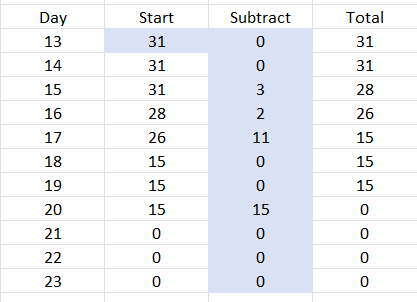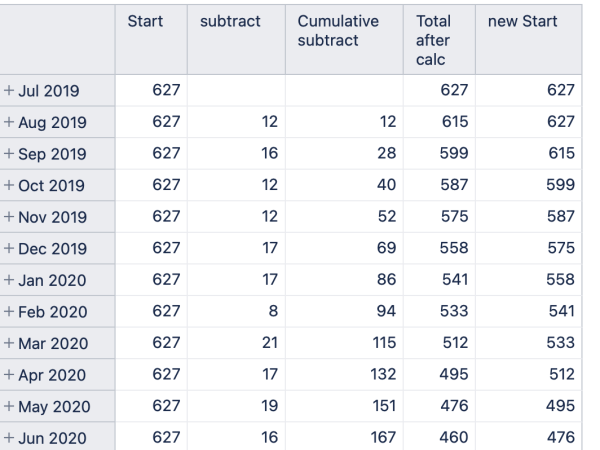# Generate a measure that can operate on itself

Hello, I have been trying to create a measure that allows me to operate with itself (I have not been successful).

The idea (as seen in the table) is that I know the data I have in the row Days 14, column Start (31) and all the numbers in the subtraction column.

The idea is to generate the following operation:

Part the operation with the first data that is known (31), then there is a condition:

Day 13:
If Subtraction (of the corresponding day) is 0 then the total is 31 and therefore the start of the next day is 31.

Day 14:
If Subtraction (of the corresponding day) is 0 then the total is 31 and therefore the start of the next day is 31.

Day 15:
If subtract (from corresponding day) > 0 then the total is 31 - 3 = 28, and the start of the next day is 28.

Day 16:
If subtract (from corresponding day) > 0 then the total is 28 - 2 = 26, and the start of the next day is 26.

Day 18:
If subtract (from corresponding day) = 0 then the total is 15 , and the start of the next day is 15.

So until the last valueYou might use the standard calculation “Cumulative sum” to achieve the desired result.
Please read more about standard calculations here - Standard Calculations.

In that case, the initial “Start” remains the same, but the value for “Total” is calculated by subtracting the cumulative sum of the “Subtract” from the initial “Start”.
The cumulative sum column is not retrieved by formula auto-filler because it is a report-specific calculation. Therefore you need to use exact naming copied from the calculation name.

The result might be like this.The expression for the “Total after calc” is the following.

``````[Measures].[Start]
-
[Measures].[Cumulative subtract]
``````

Since the cumulative sum already includes the primary measure’s current value, I added the “new Start” with the following calculation.

``````[Measures].[Start]
-
[Measures].[Cumulative subtract]
+
[Measures].[subtract]
``````

Regards,
Oskars / support@eazyBI.com